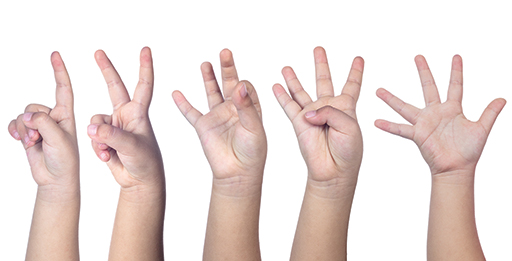Teaching mathematics

Start this free course now. Just create an account and sign in. Enrol and complete the course for a free statement of participation or digital badge if available.

Free course

# Week 1: Teaching and learning: whole numbers and decimals

## IntroductionFigure 1 A child counting from one to five

This course focuses on teaching and learning middle school mathematics. However, this week starts at the beginning by considering how children learn numbers and develop number sense (Figure 1). This underpins their later learning about the number system, and most other aspects of mathematics. As you can imagine, if the foundation for number sense is not securely developed, this will lead to later difficulties in learning mathematics.

It is important that the procedures for calculating with number (i.e. methods of doing arithmetic) are underpinned by an understanding of how those procedures work. This is known as procedural understanding. It is also important that the underlying mathematical concepts of number are understood. This is known as conceptual understanding.

Richard Skemp, the late educational psychologist, described two different ways to learn about and understand mathematics (Skemp, 1973). The procedural and conceptual understanding described above helps learners to understand how and why mathematical processes work. Skemp termed this relational understanding. On the other hand, learners may learn mathematics as several methods given them to obtain correct answers, which Skemp termed instrumental understanding. But, unless they have a very good memory for methods, they will struggle to remember the methods and the appropriate contexts for their use.

By now you will probably have concluded that the authors of this course favour relational understanding over instrumental understanding and the promotion of careful teaching which develops learners’ deep understanding of mathematics.

Week 1 of the course starts by reviewing the procedures and concepts of number operations that have been introduced to leaners before age 9 or 10. This week contains more reading than weeks 2 – 8, and less activity. It is intended to introduce you briefly to the actions, language and written methods that your learners will be familiar with.

TM_1